Follow

# Ground Sampling Distance (GSD) in Photogrammetry

## What is Ground Sampling Distance (GSD)?

Ground Sampling Distance (GSD) is the linear distance between the centers of two consecutive pixels as measured in the ground it represents.

Before diving deep, take a few seconds to study the following visualization: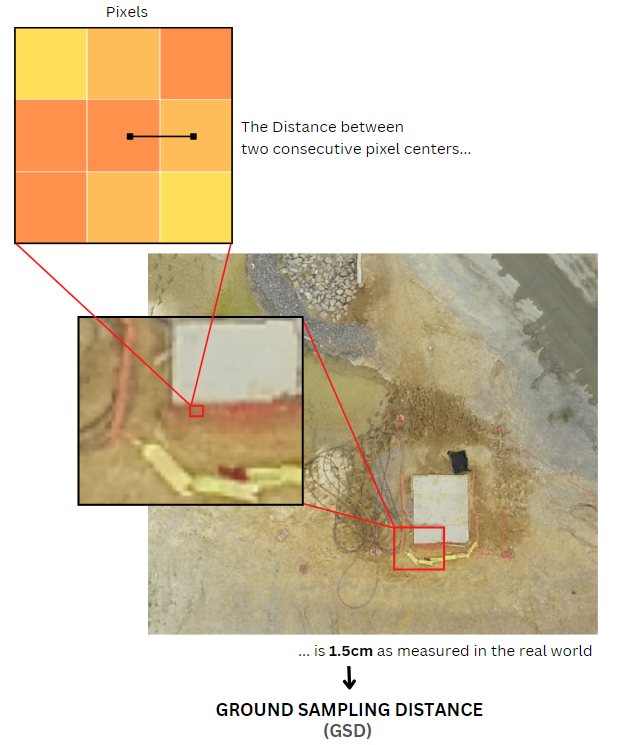## What are Pixels?

Pixels, short for “picture elements”, are the smallest addressable units found in an image. A raster image consists of hundreds to thousands to millions of pixels. More pixels per square unit mean more unique details are present.

## Pixels representing the real world

Compiling a considerable amount of pixels generate an image. Images can represent anything. In this case, we’ll be talking about images from cameras capturing the real world.

Cameras gather information from the light they receive and store those information as pixels via image sensors. This means cameras capture and keep permanent records of real-world data.

### Can we accurately make measurements using images?

Images are not perfect.  Distortions are expected due to the nature of the camera lens, orientation of the camera, etc. This hinders us from making accurate measurements. Fortunately, orthorectification of images helps us correct these distortions so that reliable measurements can be made; but even an orthorectified image has its limitations... enter GSD.

## Ground Sampling Distance (GSD)

GSD is the linear distance between the centers of two consecutive pixels as measured in the ground it represents. The larger the pixels are, the larger the linear distance/GSD is--they have direct correlation.

Since pixels are the smallest addressable units found in an image, it means that you cannot measure distance shorter than the GSD.  Say you have an image with a 1.5cm GSD, 1.5cm will be the smallest distance you will be able to perceive from the same image.

## How is GSD calculated?

Geometry tells us that similar triangles' angles are congruent, and corresponding sides are equal in proportion.  When cameras are capturing images, we can observe the following similar triangles: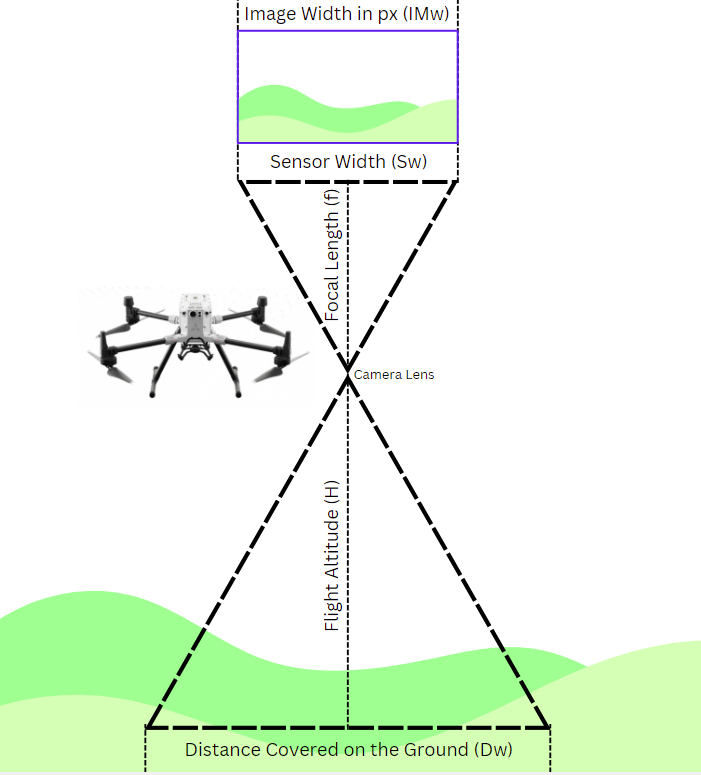With similar triangles in mind, we can say that: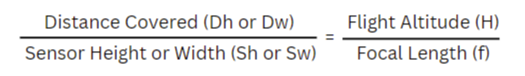We can correctly calculate the GSD using the above formula. Remember that the distance covered can be calculated by multiplying the GSD to the number of pixels. Using the image width as an example: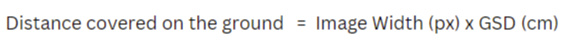Since images are usually not perfectly square, the assumed GSD of an image is the larger value (worse case). This is why we are considering only the width in this case, as image widths are usually larger than heights

With a couple of transposition and isolation, the GSD Formula can be simplified as: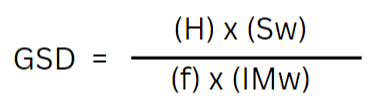Focal Length and Sensor Width/Height are usually expressed in millimeters, while flight height is expressed in meters. We need to standardize the units to get an accurate answer (e.g. convert all to centimeters). Image Width or Height are always in px, thus no conversion is needed for those values.

## Why is GSD important?

GSD tells you a lot about a given image. It tells you how detailed (or blurry) an image is; it gives you an idea how high the camera is flying; and it also gives you the accuracy level an image can attain. This is very important when looking into your inputs and outputs.

So the next time you tackle aerial imagery and photogrammetry, it will be useful to ask--What’s the GSD?

Still Having Trouble?

Send us an email at support@skycatch.com!